Homework Help Question & Answers

# (4) (a) Compute the Fourier series for the function f(x) interval [-π, π]. 1-z on the (b) Compute the solution u(t, z) for the partial differential equation on the interval [0, T): 16ut = uzz with...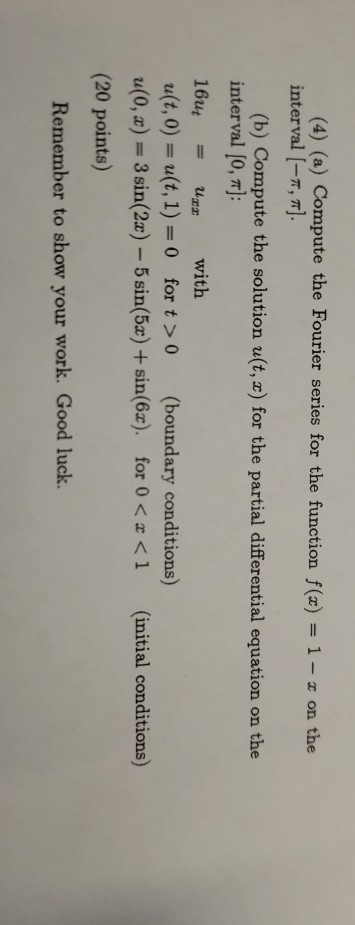(4) (a) Compute the Fourier series for the function f(x) interval [-π, π]. 1-z on the (b) Compute the solution u(t, z) for the partial differential equation on the interval [0, T): 16ut = uzz with u(t, 0)-u(t, 1) 0 for t>0 (boundary conditions) (0,) 3 sin(2a) 5 sin(5x) +sin(6x). for 0 K

#### Homework Answers

Answer #1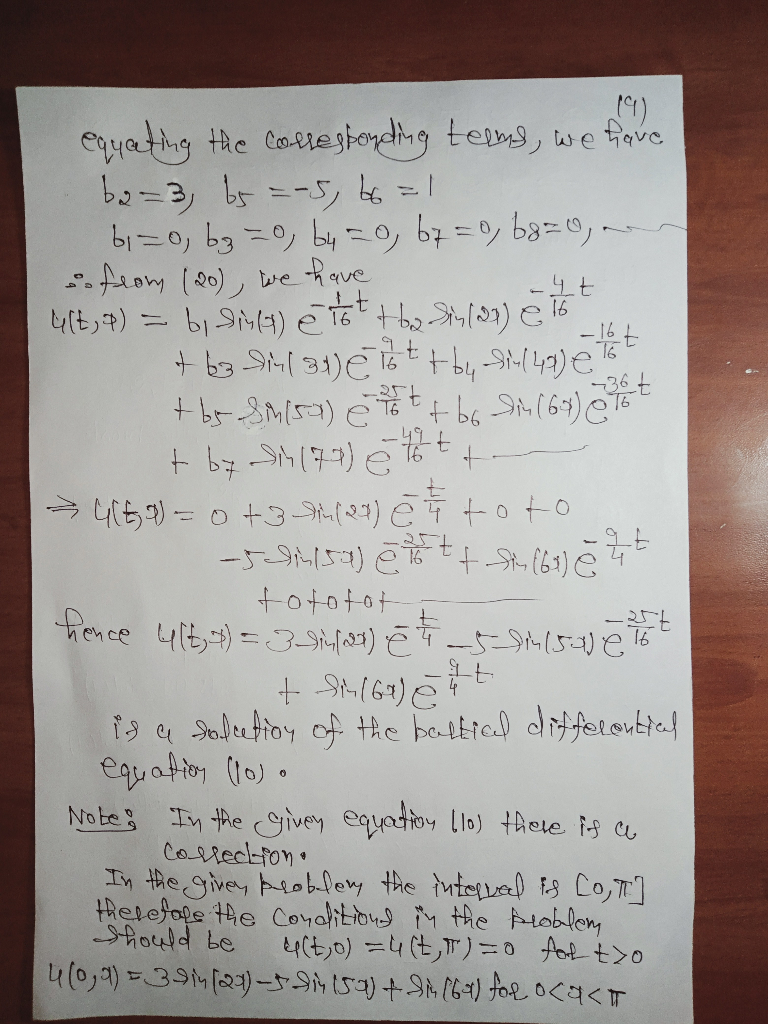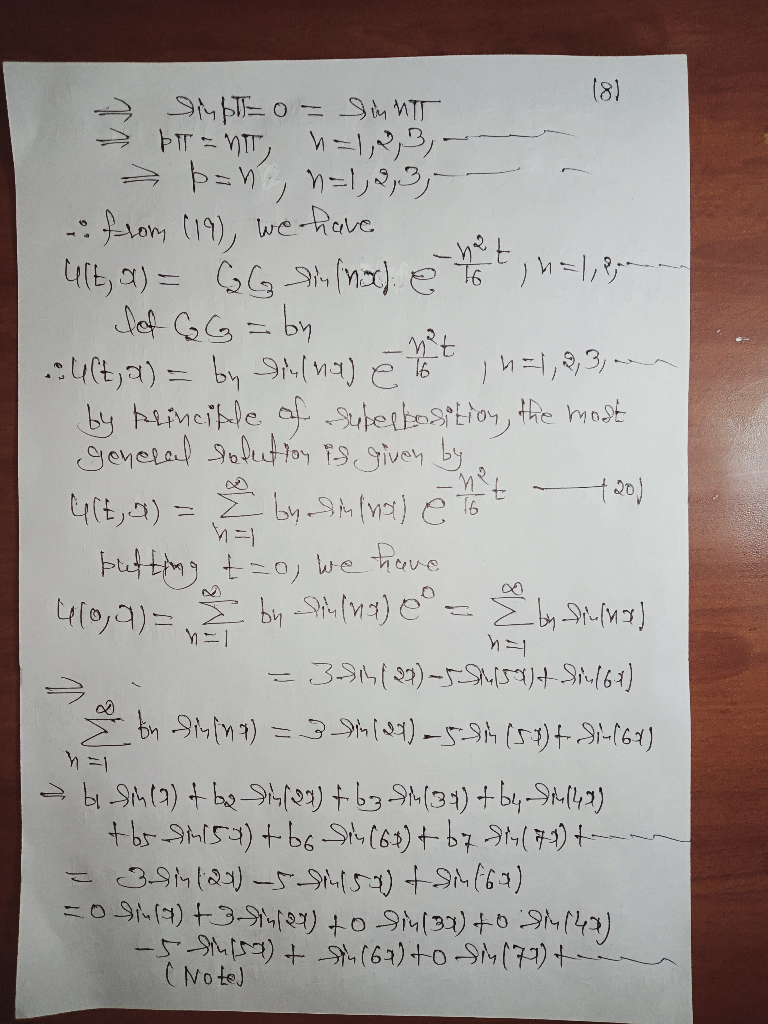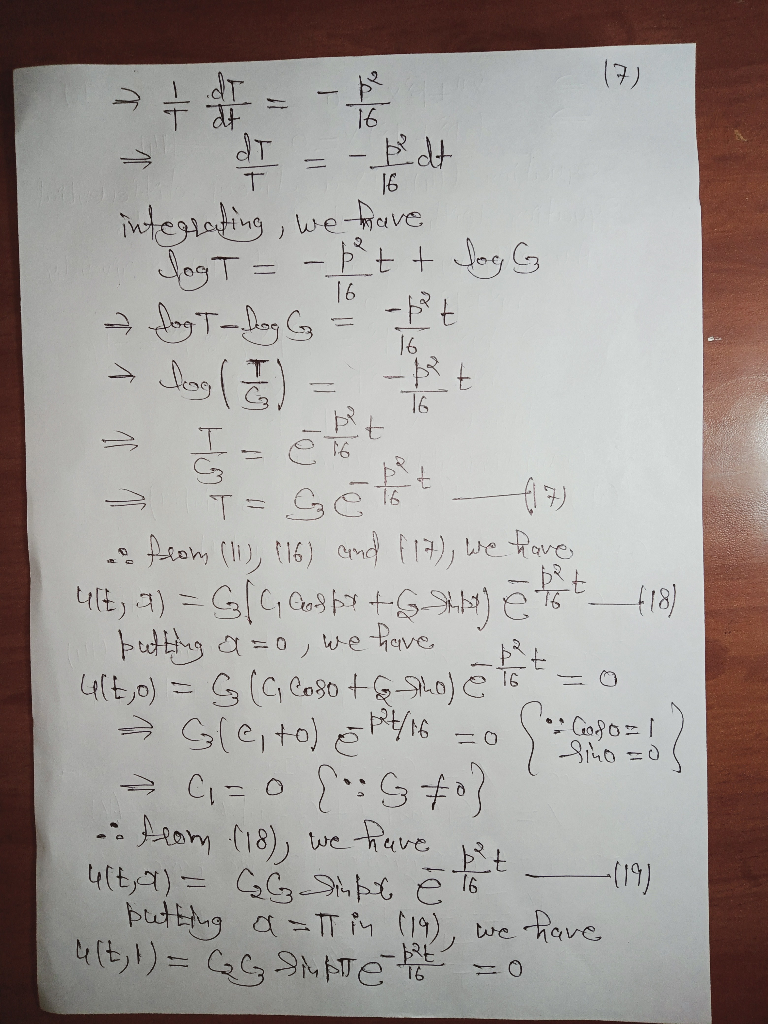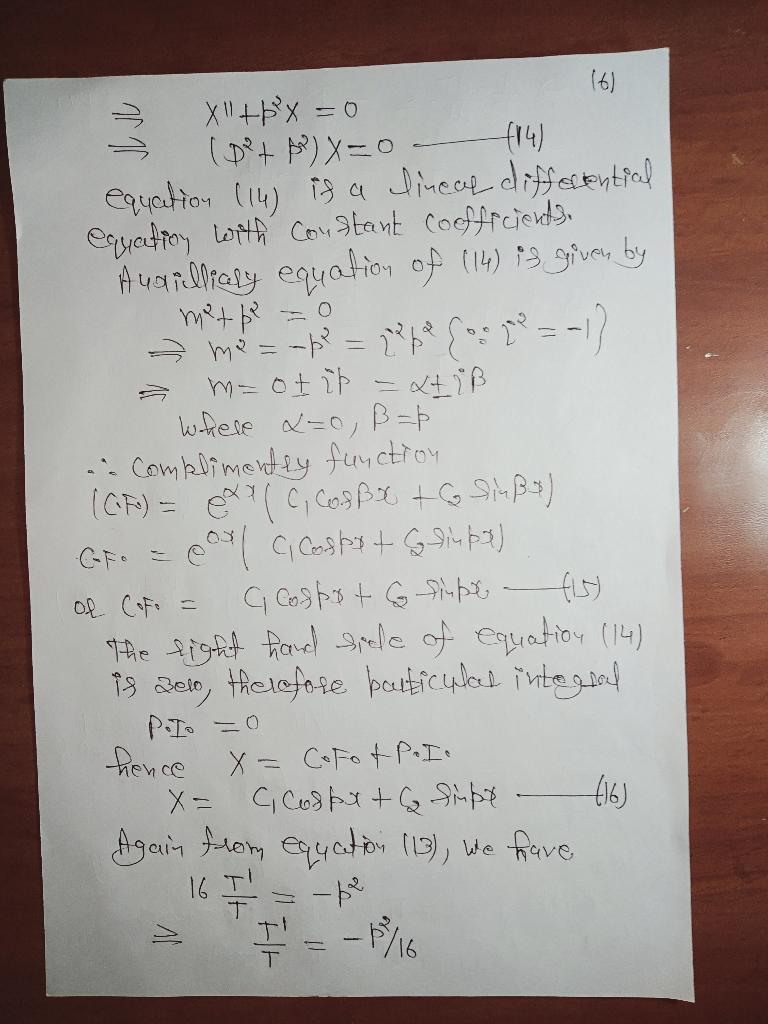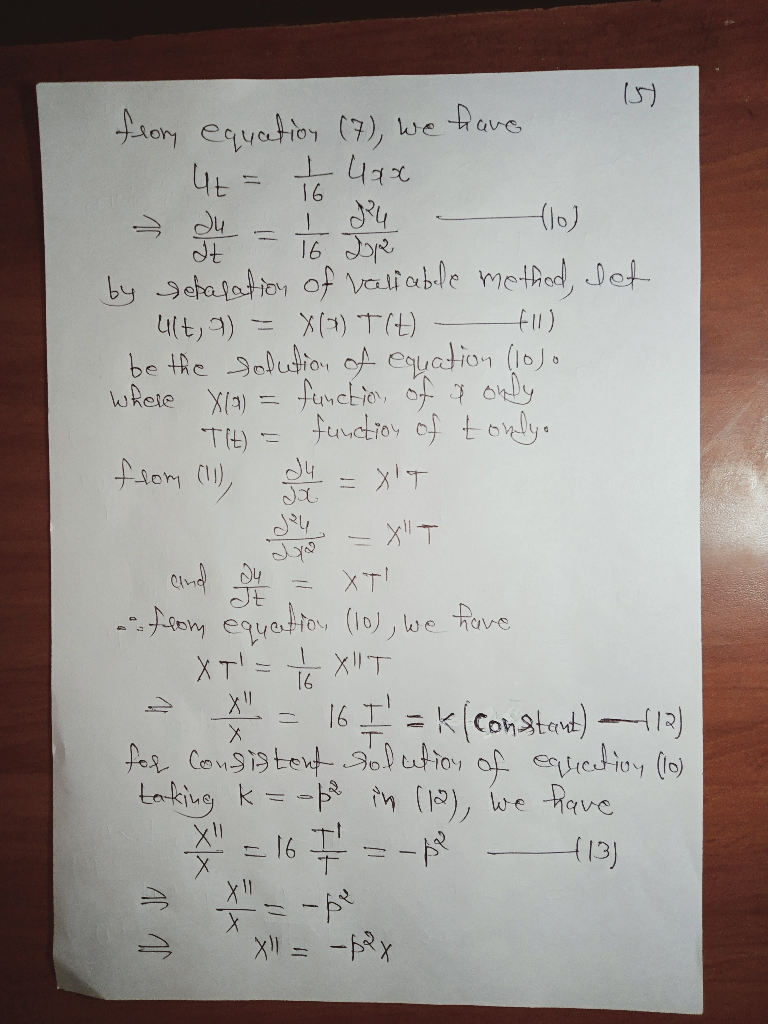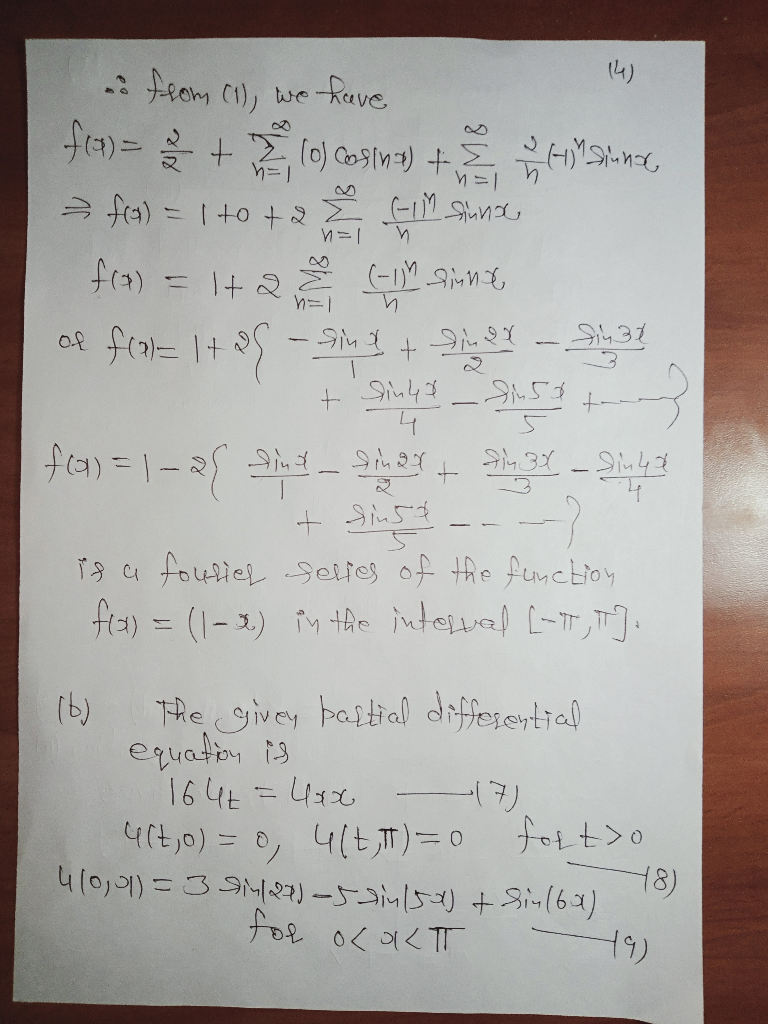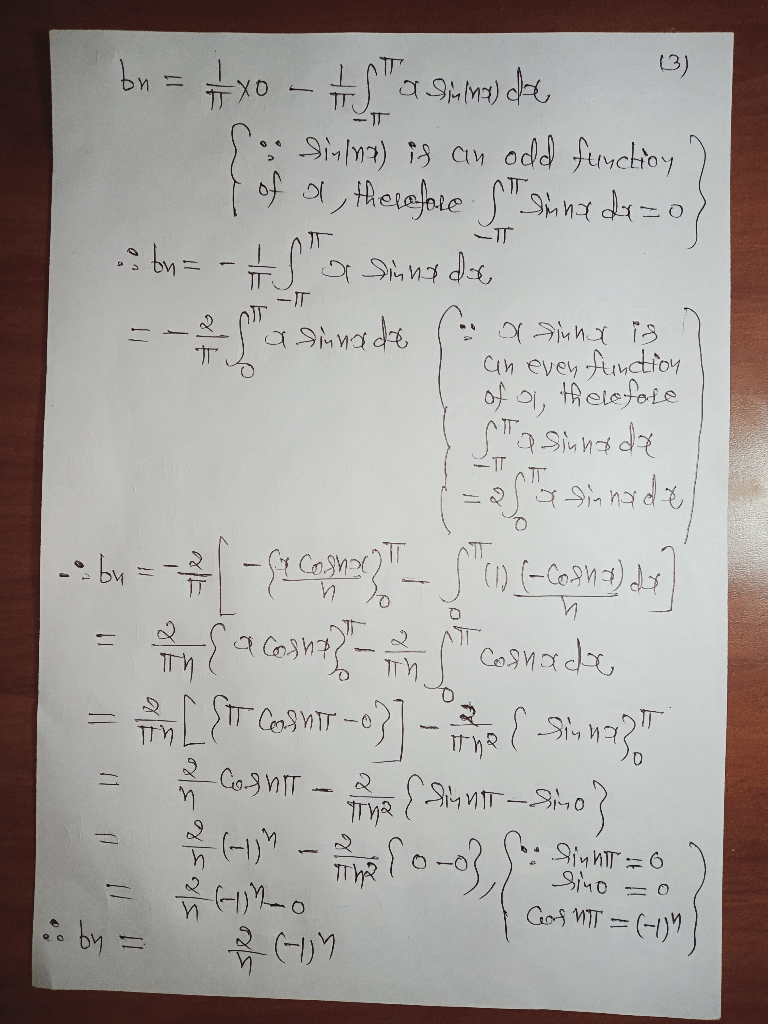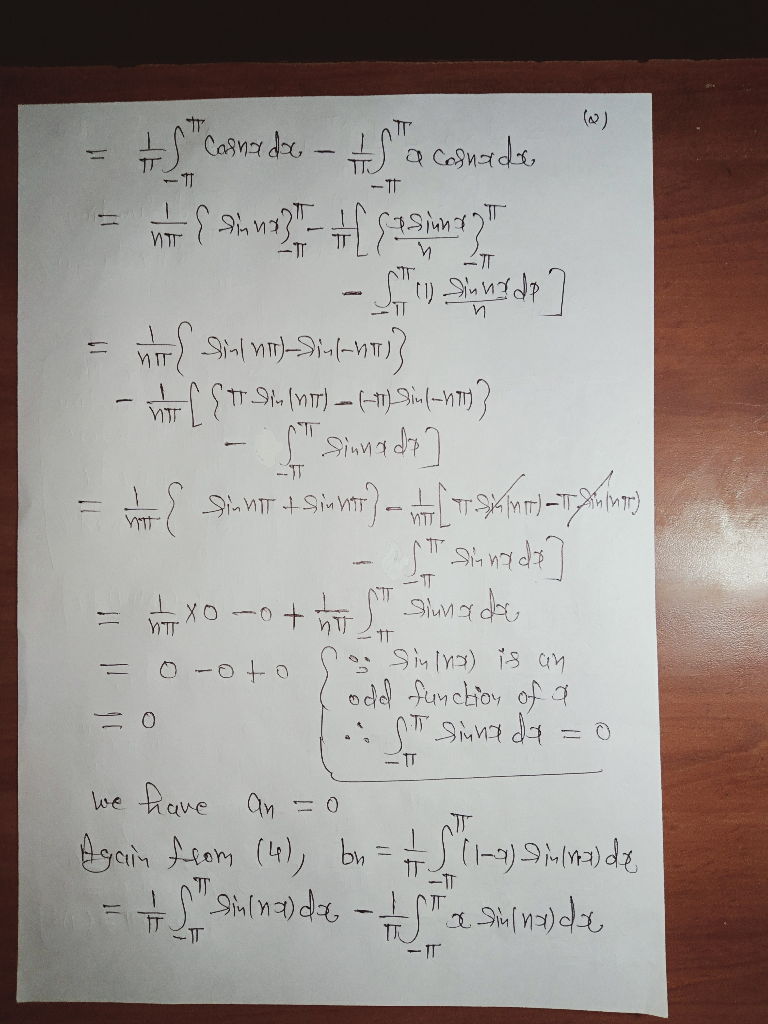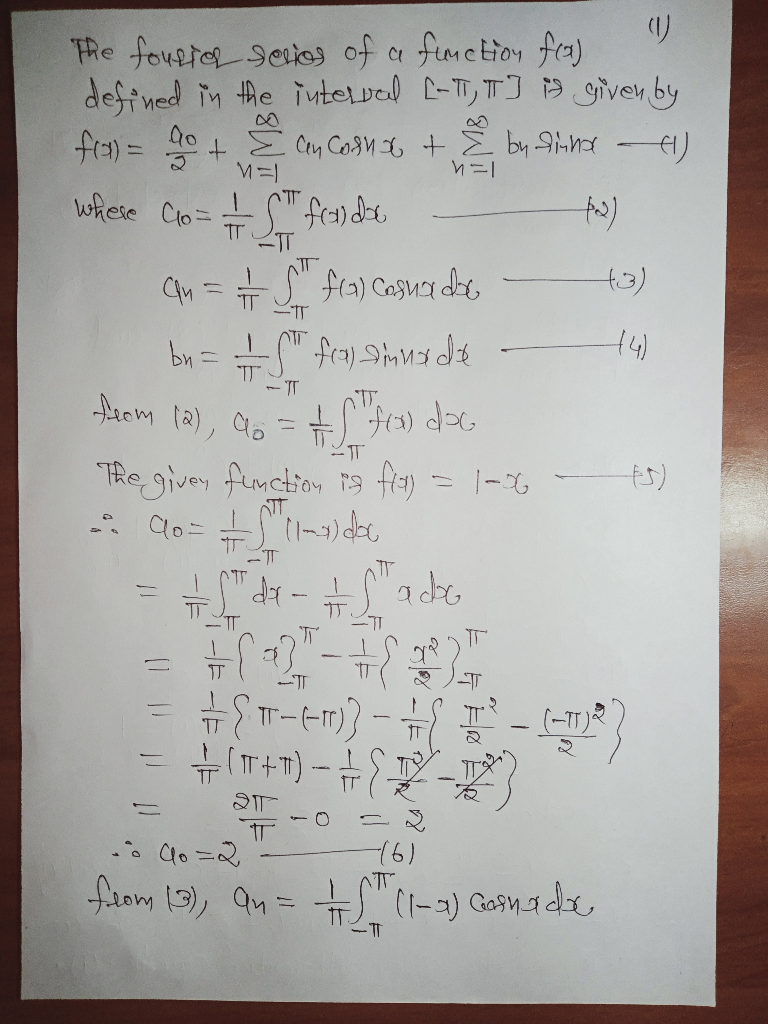Know the answer?
Your Answer:

#### Post as a guest

Your Name:

What's your source?

#### Earn Coin

Coins can be redeemed for fabulous gifts.

Not the answer you're looking for? Ask your own homework help question. Our experts will answer your question WITHIN MINUTES for Free.
Similar Homework Help Questions
• ### (4) (a) Compute the Fourier series for the function f(s)-- interval [-T, on the (b) Compute the solution u(t,a) for the partial differential equation on the interval [o, ) luWith u(t, 0) u(t,1)-0...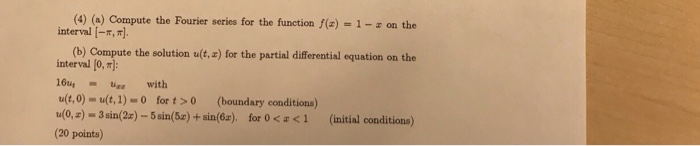(4) (a) Compute the Fourier series for the function f(s)-- interval [-T, on the (b) Compute the solution u(t,a) for the partial differential equation on the interval [o, ) luWith u(t, 0) u(t,1)-0 for t>0 (boundary conditions) u(o,z)-3 sin(2x)-5 sin(5z) + sin(6z), for O < < 1 (initial conditions) (20 points) (4) (a) Compute the Fourier series for the function f(s)-- interval [-T, on the (b) Compute the solution u(t,a) for the partial differential equation on the interval [o, )...

• ### subject is differential equations please hurry up (5) (12 poins)(a) Compute the sine series for the function f such that f(z) r(4-z) on the interval l0,4 (b) Compute the solution u(z,t) for the pa...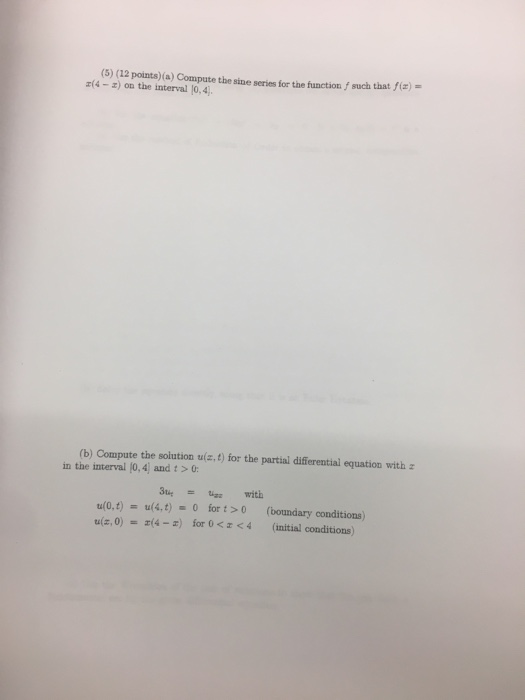subject is differential equations please hurry up (5) (12 poins)(a) Compute the sine series for the function f such that f(z) r(4-z) on the interval l0,4 (b) Compute the solution u(z,t) for the partial differential equation with z in the interval (0,4) and t > 0: 3tit tigr with u(0,t) = u(4,t)-0 for t > 0 u(z, 0)(4-a) for 0 <4 (boundary conditions) (initial conditions) (5) (12 poins)(a) Compute the sine series for the function f such that f(z) r(4-z)...

• ### 5. please help ASAP (5) (12 points)(a) Compute the sine series for the function f such that fiar) - (4- on the interval 10,4) b) Compute the solution tu(E, 2) for the partial differential equation...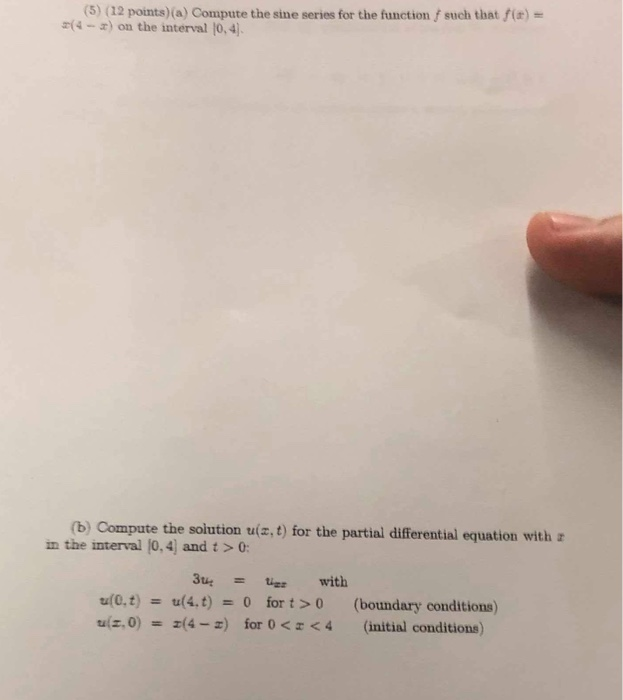5. please help ASAP (5) (12 points)(a) Compute the sine series for the function f such that fiar) - (4- on the interval 10,4) b) Compute the solution tu(E, 2) for the partial differential equation with a in the interval [0,4] and t >0 3t u(4. t) with tr(0,t) for t > 0 (boundary conditions) 0 u z, 0) (4-z for 0 <4 (initial conditions) (5) (12 points)(a) Compute the sine series for the function f such that fiar) -...

• ### Consider the partial differential equation together with the boundary conditions u(0, t) 0 and u(1,t)0 for t20 and the initial condition u(z,0) = z(1-2) for 0 < x < 1. (a) If n is a positive in...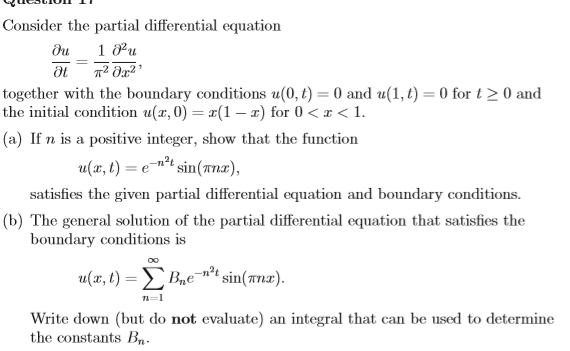Consider the partial differential equation together with the boundary conditions u(0, t) 0 and u(1,t)0 for t20 and the initial condition u(z,0) = z(1-2) for 0 < x < 1. (a) If n is a positive integer, show that the function , sin(x), satisfies the given partial differential equation and boundary conditions. (b) The general solution of the partial differential equation that satisfies the boundary conditions is Write down (but do not evaluate) an integral that can be used to...

• ### 1. Consider the Partial Differential Equation ot u(0,t) = u(r, t) = 0 a(x, 0)-x (Y), sin (! We know the general solution to the Basic Heat Equation is u(z,t)-Σ b e ). n= 1 (b) Find the unique solutio...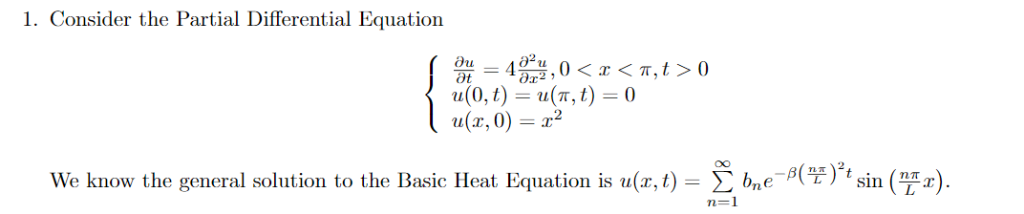1. Consider the Partial Differential Equation ot u(0,t) = u(r, t) = 0 a(x, 0)-x (Y), sin (! We know the general solution to the Basic Heat Equation is u(z,t)-Σ b e ). n= 1 (b) Find the unique solution that satisfies the given initial condition ur, 0) -2. (Hint: bn is given by the Fourier Coefficients-f(z),sin(Y- UsefulFormulas/Facts for PDEs/Fourier Series 1)2 (TiT) » x sin aL(1)1 a24(부) (TiT) 1)+1 0 1. Consider the Partial Differential Equation ot u(0,t) =...

• ### Problem 1. Consider the nonhomogeneous heat equation for u,t) ut = uzz + sin(2x), 0<x<π, t>0 subject to the nonhomogeneous boundary conditions u(0, t) t > 0 u(n, t) = 0, 1, - and the...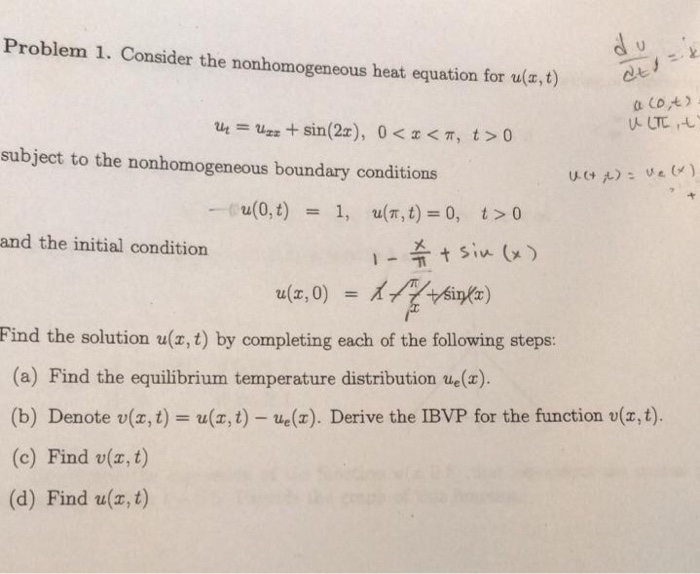Problem 1. Consider the nonhomogeneous heat equation for u,t) ut = uzz + sin(2x), 0<x<π, t>0 subject to the nonhomogeneous boundary conditions u(0, t) t > 0 u(n, t) = 0, 1, - and the initial condition Lee) Find the solution u(z, t) by completing each of the following steps: (a) Find the equilibrium temperature distribution ue(x). (b) Denote v(x, t) u(a, t) - e(). Derive the IBVP for the function v(x,t). (c) Find v(x, t) (d) Find u(, t)...

• ### The function u(x, t) satisfies the partial differential equation with the boundary conditions u(0,t) = 0...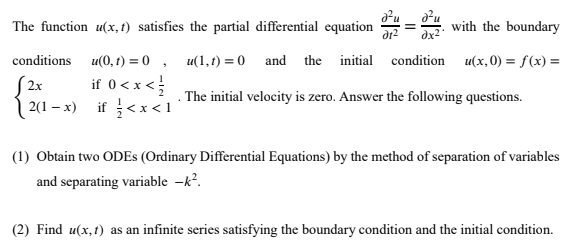The function u(x, t) satisfies the partial differential equation with the boundary conditions u(0,t) = 0 , u(1,t) = 0 and the initial condition u(x,0) = f(x) = 2x if 0<x<} 2(1 – x) if}<x< 1 . The initial velocity is zero. Answer the following questions. (1) Obtain two ODES (Ordinary Differential Equations) by the method of separation of variables and separating variable -k? (2) Find u(x, t) as an infinite series satisfying the boundary condition and the initial condition.

• ### subject is differential equations please hurry up (5) (12 poins)(a) Compute the sine series for the...subject is differential equations please hurry up (5) (12 poins)(a) Compute the sine series for the function f such that f(z) r(4-z) on the interval l0,4 (b) Compute the solution u(z,t) for the partial differential equation with z in the interval (0,4) and t > 0: 3tit tigr with u(0,t) = u(4,t)-0 for t > 0 u(z, 0)(4-a) for 0 <4 (boundary conditions) (initial conditions)

• ### 1. If Ea) 2. The Fourier series expansion of the function f() which is defined over one period by , 1<zc2 is f(...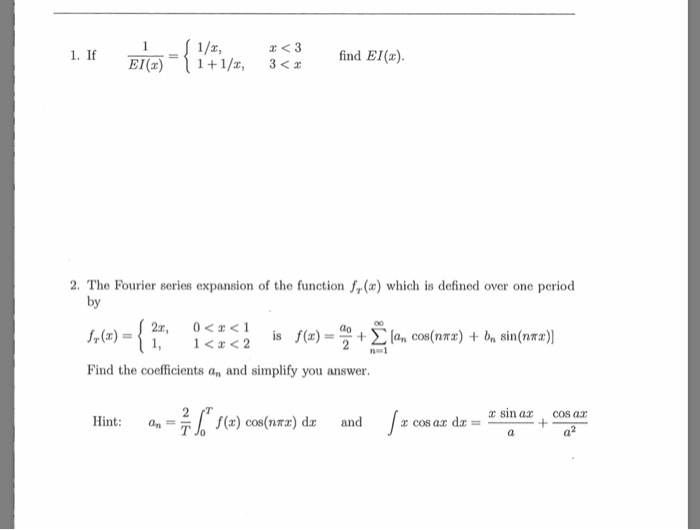1. If Ea) 2. The Fourier series expansion of the function f() which is defined over one period by , 1<zc2 is f(z) = ao + Find the coefficients an and simplify you answer. 1 z sin ax cos ar Jzcos az dz = Hint: f(x) cos(n") dz and a.-Th 3. The propagation of waves along a particular string is governed by the following bound- ary value problem u(0,t) 0 ue(8,t)0 u(x,0) = f(x) u(x,0) g(x) Use the separation of...

• ### (1 point) Suppose you're given the following Fourier coefficients for a function on the interval [-π, π : ao = 2, ak = 0 for k 2 i, and for k > 1. Find the following Fourier approximations to...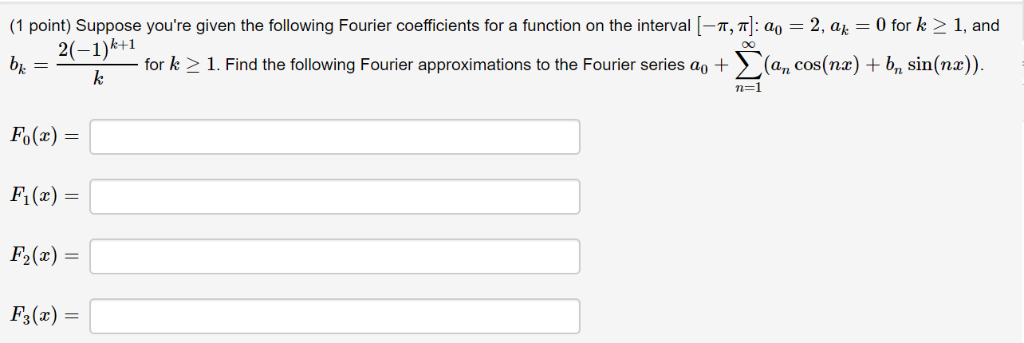(1 point) Suppose you're given the following Fourier coefficients for a function on the interval [-π, π : ao = 2, ak = 0 for k 2 i, and for k > 1. Find the following Fourier approximations to the Fourier series a0 + 〉 ,(an cos(nz) + bn sin(nx)) bk = F, (z) = F,(z) = Fs(x) (1 point) Suppose you're given the following Fourier coefficients for a function on the interval [-π, π : ao = 2, ak...

Free Homework App

Scan Your Homework
to Get Instant Free Answers
Need Online Homework Help?

Get Answers For Free
Most questions answered within 3 hours.